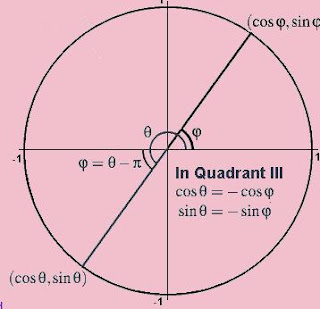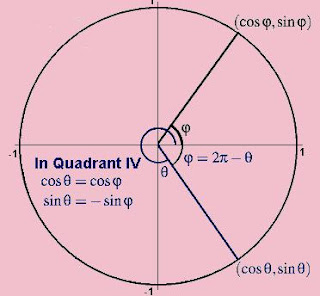Saturday, September 26, 2009

Reference Angle

Reference angles are used to determine the values of the trigonometric functions in the second, third and fourth quadrants, in particular, for the "nice" angles. The reference angle for an angle θ is the smallest angle φ from the (positive or negative) x-axis to the terminal ray of the angle θ.

For an angle θ in the second quadrant the reference angle φ is the remaining angle needed to complete a straight angle, that is, π radians or 180°. Thus θ + φ = π or θ + φ = 180°, and so

φ = π - θ or φ = 180° - θ.For an angle θ in the third quadrant the reference angle φ is the angle that must be subtracted from θ to leave a straight angle, that is, π radians or 180°. Thus θ - φ = π or θ - φ = 180°, and so

φ = θ - π or φ = θ - 180°